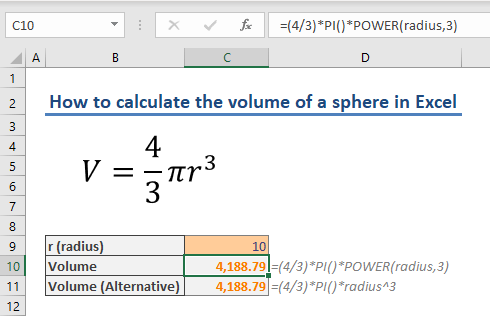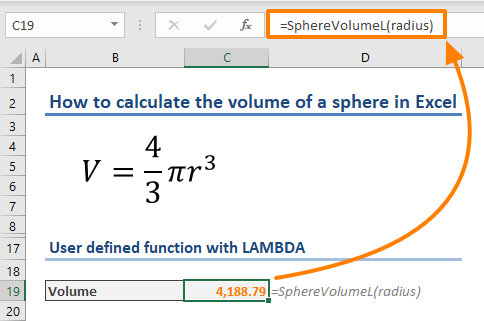In this guide, we're going to show you how to calculate the volume of a sphere in Excel.

The formula for finding the volume of a sphere is the following:The number π is a mathematical constant defined as the ratio of a circle's circumference to its diameter. It is approximately equal to 3.14159, but the constant is stored as a function in Excel - see PI().

The number r represents the radius of the sphere. You need to calculate the cube of the radius which can be calculated with either the POWER function or the exponential operator (^).

or

All you need to is using one of the formulas above and supply a value for the radius. In the following screenshot, you can see that both functions return same result. Note that the cell C9 in our example is named “radius”.## Creating a custom function to calculate the volume of a sphere

If you need to use this function frequently, custom functions - also known as user defined functions (UDF) - can be helpful.

As the name suggests, custom functions are functions you can build in Excel with your parameters. Since there are no built-in functions to calculate the volume of a sphere, you can create this as a custom function.

At the time of writing this article, there are 2 ways of creating UDFs:

1. VBA (Visual Basic for Applications)
2. LAMBDA Function (requires Microsoft 365 subscription)

### Using VBA to calculate the volume of a sphere

1. Press the Alt + F11 key combination to open the VBA window
3. Copy the following code into the text area of the module
```Public Function SphereVolumeVBA(radius)
SphereVolumeVBA = (4 / 3) * Application.WorksheetFunction.Pi() * (radius ^ 3)
End Function```

You can use SphereVolumeVBA() formula right away. This formula can calculate the volume of a sphere quickly anywhere in your workbook.Remember to save the file in XLSM (Macro enabled Excel Spreadsheet) format. Otherwise, you will lose the code in the VBA window.

You can check our User Defined Functions in Excel article for detailed information about user defined functions.

### Using LAMBDA Function

As of Jan 2021, the LAMBDA is only available for Microsoft 365 subscribers. This special function can convert named ranges into user defined functions.

Let’s see how you can create a custom function with the LAMBDA.

1. Click Define Name in Formulas tab in the Ribbon
2. Enter a name for your formula (our name: SphereVolumeL)
3. Enter the following formula into the Refers to section: